# Product and Quotient rule

## Summary and examples

There is a number of standard functions with known derivatives. For example, the derivative of the function:is: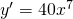It is convenient and advisable to have these standard derivatives ready so that you do not have to look them up all the time.
There are also functions you want to differentiate which are not in the table of standard functions. There are two important types.

1. The product of two functions: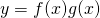In this case we make use of the so-called product rule.

If:then: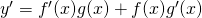2. The quotient of two functions:In this case we make use of the so-called quotient rule.

If:then:In the following examples we apply the product and quotient rule.

##### Example 1

Differentiate: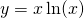In this case we have: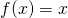and: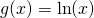and thus: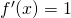and:Applying the product rule we get: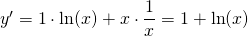.

##### Example 2

Differentiate: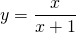In this case we have to apply the quotient rule with:and: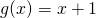Then we get: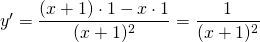##### Example 3

Differentiate:Here we have to use the quotient rule with: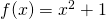and: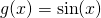Because: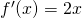and: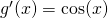the result is: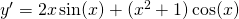##### Example 4

Differentiate: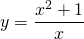Here we have:and:so:and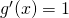Then the quotient rule yields: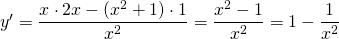This result can also be obtained without using the product rule. We can write: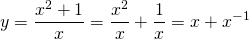We can differentiate this function using the table of standard functions, with the same result.

0